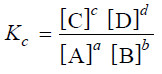# Law of Mass action

## Law of Mass action

– Two Norwegian chemists, Guldberg and Waage, studied experimentally a large number of equilibrium reactions. In 1864, they postulated a generalisation called the Law of Mass action.

– Law of Mass action states that : the rate of a chemical reaction is proportional to the active masses of the reactants.

– By the term (active mass) is meant the molar concentration i.e., number of moles per litre.

– It is expressed by enclosing the formula of the substance in square brackets.

– Thus a gas mixture containing 1 g of hydrogen (H2) and 127 g of iodine (I2) per litre has the concentrations:

[H2] = 0.5

[I2] = 0.5

## Explanation of the Law of Mass Action Based on the Molecular Collision Theory

– We assume that a chemical reaction occurs as a result of the collisions between the reacting molecules.

– Although some of these collisions are ineffective, the chemical change produced is proportional to the number of collisions actually taking place.

– Thus at a fixed temperature the rate of a reaction is determined by the number of collisions between the reactant molecules present in unit volume.

### Experiment to explain Collisions

– Let us consider four boxes of one cubic centimetre volume; containing different number of reacting molecules A and B (Fig) as follow: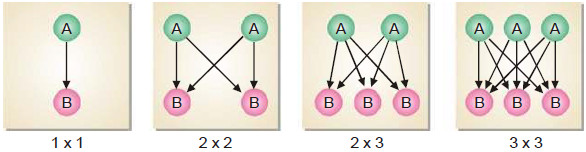– They undergo collisions to form the products C and D, the rate of reaction being governed by the number of possible collisions between them.

– In the first box there is one molecule of A and one molecule of B, and the possibilities of collision at any instant is 1 × 1 = 1.

– In the second box, the number of molecules of A and B are two each and the possibilities of collisions are 2 × 2 = 4.

– And In the third box, there are two molecules of A and three molecules of B, and the number of possibilities of collisions between A and B are increased to 3 × 2 = 6.

– In the fourth box, we have three molecules of each of A and B and the total number of collisions between them is 3 × 3 = 9.

– Thus in general, we can say that the possibilities of collisions between the reacting molecules A and B are equal to the product of the number of molecules of each species per unit volume.

– Since the rate of reaction is determined by molecular impacts, it is proportional to moles per unit volume i.e., molar concentration.

– Thus we can write

Rate of reaction ∝ [A] [B]

= k [A] [B]

In a reaction, A + B + C ⎯⎯→ the number of collisions between A, B and C depends on the concentration of each reactant (Fig)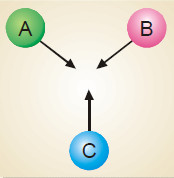Therefore,

Rate of reaction ∝ [A] [B] [C] = k [A] [B] [C]

From the above considerations: it stands proved that the rate of a reaction is proportional to the molar concentrations of the reactants.

## Equilibrium constant :Equilibrium law

– Let us consider a general reaction

A + B ↔ C + D

– and let [A], [B], [C] and [D] represent the molar concentrations of A, B, C and D at the equilibrium point.

– According to the Law of Mass action.

Rate of forward reaction ∝ [A] [B] = k1 [A] [B]

Rate of reverse reaction ∝ [C][D] = k2 [C][D]

– where k1 and k2 are rate constants for the forward and reverse reactions.

– At equilibrium, rate of forward reaction = rate of reverse reaction.

Therefore,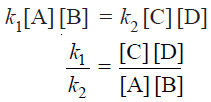– At any specific temperature k1/k2 is constant since both k1 and k2 are constants.

– The ratio k1/k2 is called Equilibrium constant and is represented by the symbol Kc, or simply k.

– The subscript ‘c’ indicates that the value is in terms of concentrations of reactants and products.

– The equation (1) may be written as :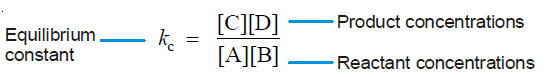– This equation is known as the Equilibrium constant expression or Equilibrium law

– The general reaction may be written as:

aA + bB ↔ cC + dD

– where a, b, c and d are numerical quotients of the substance, A, B, C and D respectively.

– The equilibrium constant expression is: